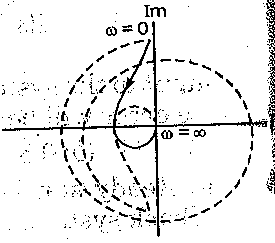# For the transfer function G(s)H(s)=1/s(s+1)(s+0.5) the phase cross-over frequency is

4

Explanation :
No Explanation available for this question

# What is the unit step response of a unity feedback control system having, forward path transfer function G(s)=80/ s(s + 18)

1.  Overdamped

2.  Critically damped

3.  Underdamped

4.  Undamped oscillatory

4

Undamped oscillatory

Explanation :
No Explanation available for this question

# The correct sequence of steps needed to improve ,system stability is

1.  insert derivative action, use negative fee back reduce gain

2.  reduce gain, use negative feedback, insert derivation action

3.  reduce gain, insert derivation action, use negative feedback

4.  use negative feedback, reduce gain, insert derivation action

4

use negative feedback, reduce gain, insert derivation action

Explanation :
No Explanation available for this question

# Consider the following polynomials : 1. s4 + 7s3 + 17s2 + 17s + 6. 2. s4 + 11s3 + 41s2 + 61s + 30. 3. s4 + s3 + 2s2 + 3s + 2. Among these polynomials, those which are Hurwitz are

1.  1 and 3

2.  2 and 3

3.  1 and 2

4.  1, 2 and 3

4

1 and 2

Explanation :
No Explanation available for this question

# The open-loop transfer function of a unity feedback control system is G (s) H (s)=30/ s (s +1) (s + T) where T is a variable parameter. The closed-loop system will be stable for all value use of

1.  T>0

2.  0 < T < 3

3.  T > 5

4.  3

4

T > 5

Explanation :
No Explanation available for this question

# The open loop transfer function of a system is G(s)H(s)=K(1 + s)2 /s3 he Nyquist plot for this system. is

1.

2.

3.

4.

4Explanation :
No Explanation available for this question

# The number of roots of the equation 2s4 + s3 + 3s2 + 5s + 7 = 0 that lie in the right half of s plane is

1.  zero

2.  one

3.  two

4.  three

4

two

Explanation :
No Explanation available for this question

# The feedback system with characteristic equation s4 + 20Ks3 + 5s2 +10s + 15 = 0 is

1.  stable for all value of K

2.  stable for positive value of K

3.  stable for ∞ > K > 7.0

4.  unstable for all values of K

4

unstable for all values of K

Explanation :
No Explanation available for this question

# consider a unity feedback system having an open-loop transfer function G(jω)=K/ j(jo.2ω+1) ( j0.05ω +1) For K =1, GM = 28db.:When GM at 20 dB, K will be equal to

1.  2

2.  2.5

3.  4

4.  5

4

2.5

Explanation :
No Explanation available for this question

1.  1

2.  2

3.  3

4.  4

4